NCERT Solutions for Class 6 Maths Chapter 3 Playing with Numbers Ex 3.6

# NCERT Solutions for Class 6 Maths Chapter 3 Playing with Numbers Ex 3.6

## NCERT Solutions for Class 6 Maths Chapter 3 Playing with Numbers Ex 3.6

NCERT Solutions for Class 6 Maths Chapter 3 Playing with Numbers Ex 3.6 are the part of NCERT Solutions for Class 6 Maths. Here you can find the NCERT Solutions for Class 6 Maths Chapter 3 Playing with Numbers Ex 3.6.

### Ex 3.6 Class 6 Maths Question 1.

Find the HCF of the following numbers:
(a) 18, 48
(b) 30, 42
(c) 18, 60
(d) 27, 63
(e) 36, 84
(f) 34, 102
(g) 70, 105, 175
(h) 91, 112, 49
(i) 18, 54, 81
(j) 12, 45, 75

Solution:
(a) The given numbers are 18 and 48.
Prime factorisations of 18 and 48 are: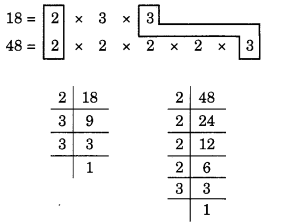Here, the common factors are 2 and 3.
Hence, the HCF = 2 × 3 = 6

(b) The given numbers are 30 and 42.
Prime factorisations of 30 and 42 are:Here, the common factors are 2 and 3.
Hence, the HCF = 2 × 3 = 6

(c) The given numbers are 18 and 60.
Prime factorisations of 18 and 60 are: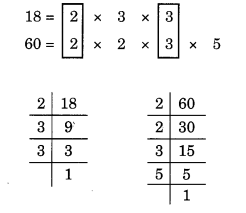Here, the common factors are 2 and 3.
Hence, the HCF of 18 and 60 = 2 × 3 = 6

(d) The given numbers are 27 and 63.
Prime factorisations of 27 and 63 are:Here, the common factor is 3 × 3.
Hence, the HCF = 3 × 3 = 9

(e) The given numbers are 36 and 84.
Prime factorisations of 36 and 84 are: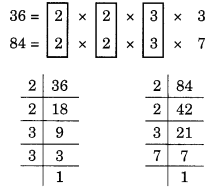Here, the common factors are 2, 2 and 3.
Hence, the HCF = 2 × 2 × 3 = 12

(f) The given numbers are 34 and 102.
Prime factorisations of 34 and 102 are: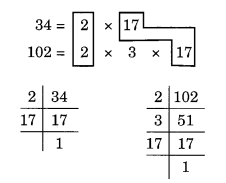Here, the common factors are 2 and 17.
Thus, the HCF = 2 × 17 = 34

(g) The given numbers are 70, 105 and 175.
Prime factorisations of 70, 105 and 175 are:Here, common factors are 5 and 7.
Hence, the HCF of 70, 105 and 175 is 5 × 7 = 35

(h) The given numbers are 91, 112 and 49.
Prime factorisations of 91, 112 and 49 are: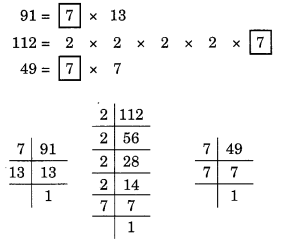Here, the common factor is 7.
Hence, the HCF = 7

(i) The given numbers are 18, 54 and 81.
Prime factorisations of 18, 54 and 81 are:Here, the common factor is 3 × 3.
Thus, the HCF = 3 × 3 = 9

(j) The given numbers are 12, 45 and 75.
Prime factorisations of 12, 45 and 75 are: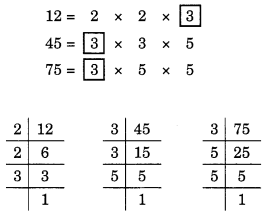Here, the common factor is 3.
Hence, the HCF = 3

### Ex 3.6 Class 6 Maths Question 2.

What is the HCF of two consecutive
(a) numbers?
(b) even numbers?
(c) odd numbers?

Solution:
(a) The common factor of two consecutive numbers is always 1.
Hence, the HCF of two consecutive numbers = 1
(b) The common factors of two consecutive even numbers are 1 and 2.
Hence, the HCF of two consecutive even numbers = 1 × 2 = 2
(c) The common factor of two consecutive odd numbers is 1.
Hence, the HCF of two consecutive odd numbers = 1

### Ex 3.6 Class 6 Maths Question 3.

HCF of co-prime numbers 4 and 15 was found as follows by factorisation:
4 = 2 × 2 and 15 = 3 × 5. Since there is no common prime factors, so HCF of 4 and 15 is 0.
Is the answer correct? If not, what is the correct HCF?

Solution:
No, the answer is not correct.
Reason: 0 is not the prime factor of any number.
1 is always the prime factor of co-prime numbers.
Hence, the correct HCF of 4 and 15 is 1.

You can also like these:

NCERT Solutions for Maths Class 7

NCERT Solutions for Maths Class 8

NCERT Solutions for Maths Class 9

NCERT Solutions for Maths Class 10

NCERT Solutions for Maths Class 11

NCERT Solutions for Maths Class 12